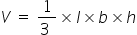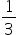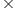Mathematics
Easy

Question

# A sign made of solid wood is in the shape of a pyramid. The base is a triangle with a base of 6 feet and a height of 4 feet. The height of the sign is 7 feet. The wood costs \$3 per cubic foot. Find the cost of the sign.

## \$168\$56\$84\$28Hint:

## The correct answer is: \$84

### Given:a base of a pyramid b =  6 feet a height of  a pyramid h = 4 feetTo find: Cost of the sign made of solid wood in the shape of a pyramid =?Solution:A sign is made up of solid wood in the shape of a pyramid.The base of a  triangle is 6 feet and the height is 4 feet.The height of the wooden sign is 7 feet.Suppose the V, h, l, and b be the volume, height, base length, and base width of the pyramid.The volume of a pyramid is given by the formula,V =647V = 56 feet3But The wood costs \$3 per cubic foot.Suppose T is the total cost of the sign. T = 563T = \$168 So the correct option is b.

First, we can calculate the base area and then multiply it by height.

### Related Questions to study#### With Turito Foundation.#### Get an Expert Advice From Turito.